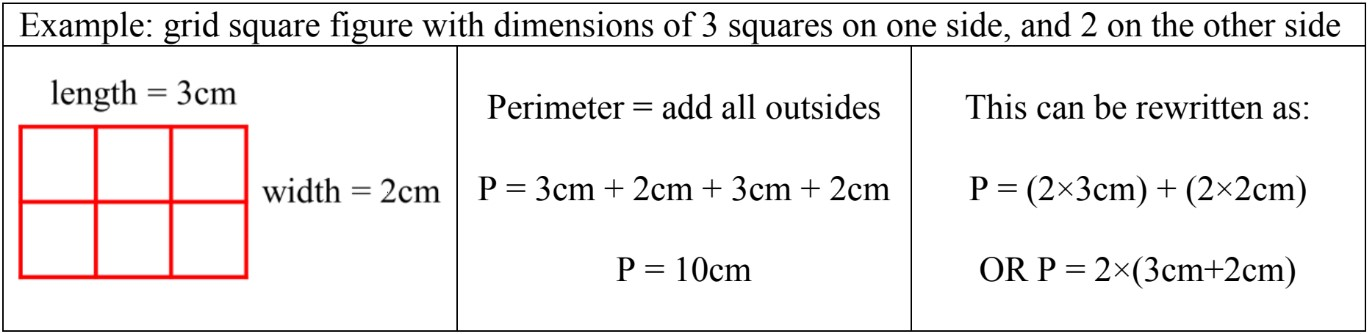# Perimeter: Grid squares and polygons#### Everything You Need in One Place

Homework problems? Exam preparation? Trying to grasp a concept or just brushing up the basics? Our extensive help & practice library have got you covered.#### Learn and Practice With Ease

Our proven video lessons ease you through problems quickly, and you get tonnes of friendly practice on questions that trip students up on tests and finals.#### Instant and Unlimited Help

Our personalized learning platform enables you to instantly find the exact walkthrough to your specific type of question. Activate unlimited help now!

0/3
##### Intros
###### Lessons
1. Introduction to Perimeter - Grid Squares and Polygons:
2. What is perimeter?
3. How to find the perimeter of polygons
4. How to find perimeter of figures with grid squares
0/15
##### Examples
###### Lessons
1. Perimeter of polygons
Calculate the perimeter of the polygon. Remember to write the units in your answer.
1.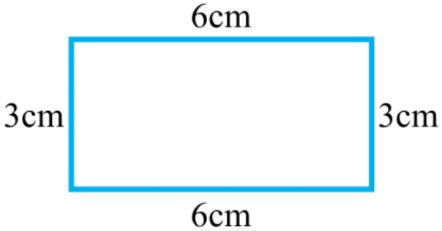2.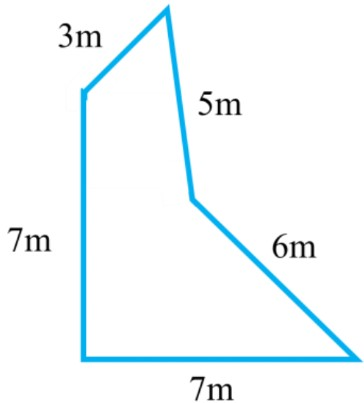3.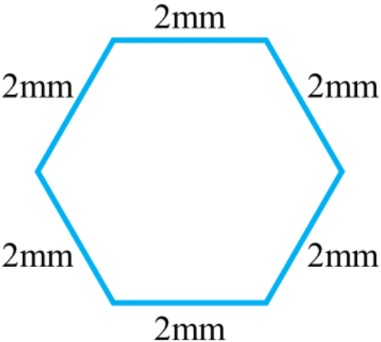2. Perimeter by tracing the outside of grid squares
Trace around the grid squares to find the perimeter (in centimeters)
1.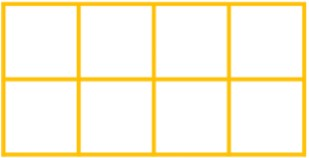2.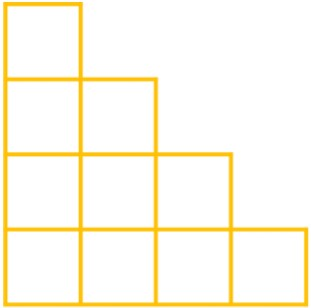3.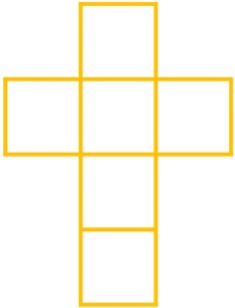3. Perimeter equations with addition or multiplication
Fill in the blanks for the rectangle made of grid squares. Complete the equations for the perimeter.
1.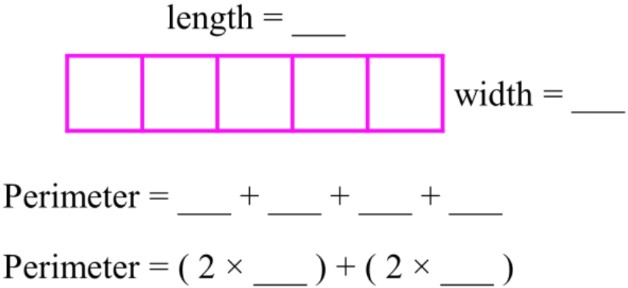2.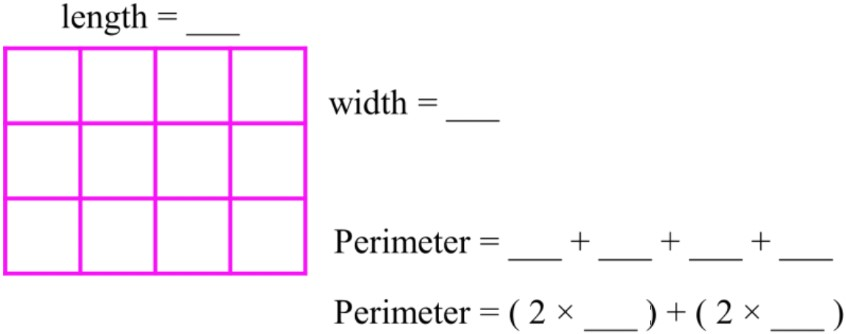3.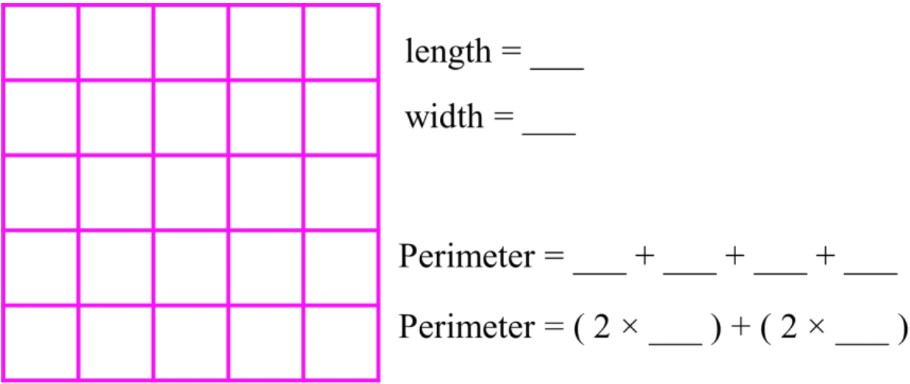4. Perimeter of shapes on grid paper
A centimeter grid paper is shown with a figure drawn on it.
1. What is the perimeter of the figure?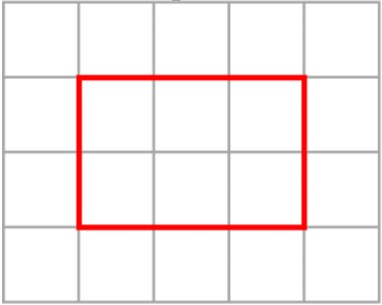2. Different shapes can have the same perimeter. Draw another rectangle with the same perimeter.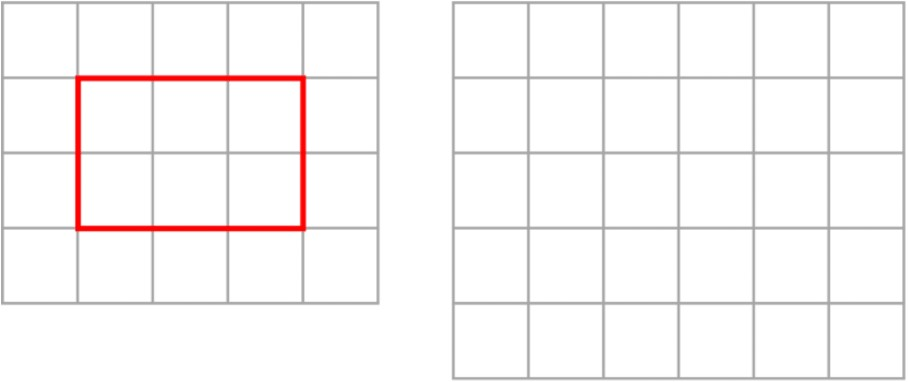3. Two figures with the same perimeter are shown below. Are they made out of the same number of grid squares? Explain why or why not.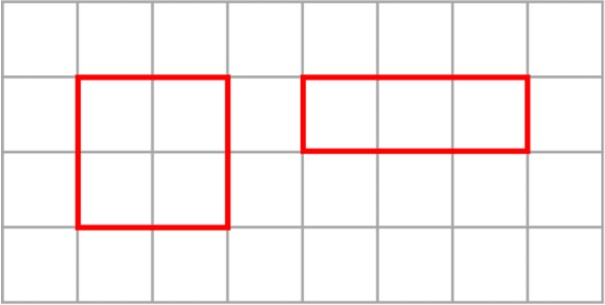5. Perimeter of polygons - word problems
Use your knowledge of polygons to calculate the perimeter.
1. What is the perimeter of a regular hexagon with 5cm on each side?
2. What is the perimeter of a rectangle with a length of 4m and a width of 9m?
3. If a regular octagon has a perimeter of 56mm, what is the length of each side?
###### Free to Join!
StudyPug is a learning help platform covering math and science from grade 4 all the way to second year university. Our video tutorials, unlimited practice problems, and step-by-step explanations provide you or your child with all the help you need to master concepts. On top of that, it's fun - with achievements, customizable avatars, and awards to keep you motivated.
• #### Easily See Your ProgressWe track the progress you've made on a topic so you know what you've done. From the course view you can easily see what topics have what and the progress you've made on them. Fill the rings to completely master that section or mouse over the icon to see more details.
• #### Make Use of Our Learning Aids###### Practice Accuracy

Get quick access to the topic you're currently learning.

See how well your practice sessions are going over time.

Stay on track with our daily recommendations.

• #### Earn Achievements as You LearnMake the most of your time as you use StudyPug to help you achieve your goals. Earn fun little badges the more you watch, practice, and use our service.
• #### Create and Customize Your AvatarPlay with our fun little avatar builder to create and customize your own avatar on StudyPug. Choose your face, eye colour, hair colour and style, and background. Unlock more options the more you use StudyPug.
###### Topic Notes

In this lesson, we will learn:

• Perimeter is the straight-line measurement of the total distance to trace around a shape
• The perimeter of a polygon can be found by adding the lengths of all sides together
• The perimeter of a figure made of grid squares is found by tracing around the outside edges only
• The formula shortcuts for the perimeter of rectangular figures made of grid squares

Notes:

• Perimeter is the measurement around the outside of a two-dimensional shape
• For a polygon (shape with straight sides only), the perimeter can be calculated by adding all the sides in the shape together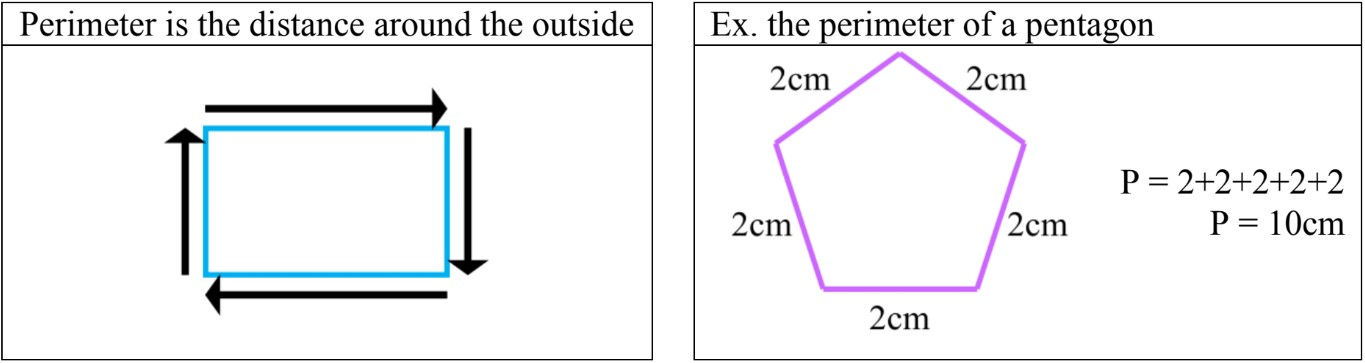• Grid squares are building blocks of figures and measure 1cm on all sides.
• To find the perimeter of a shape made of grid squares, count each edge around the OUTSIDE of the shape only.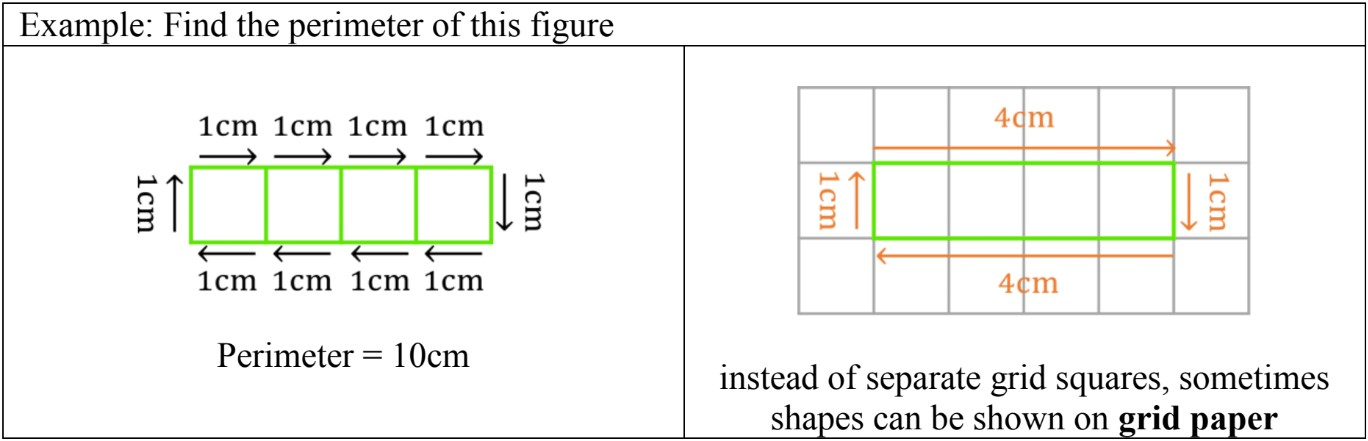• If grid squares make a rectangular figure (4-sided polygon with 2 pairs of matching sides), then a short cut can be used for finding the perimeter:
• Perimeter =2 × length + (2 × width)
• OR Perimeter =2 × (length + width)